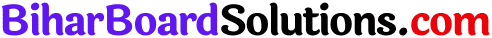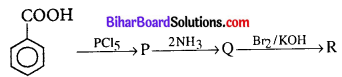# Bihar Board 12th Chemistry VVI Objective Questions Model Set 2 in English

Bihar Board 12th Chemistry Objective Questions and Answers

## Bihar Board 12th Chemistry VVI Objective Questions Model Set 2 in English

Question 1.
Nitrogen is used to fill electric bulbs because
(a) it is lighter than air
(b) it makes the bulb to glow
(c) it does not support combustion
(d) it is non-toxic
(c) it does not support combustion

Question 2.
Which of the following compounds will not give amount on heating?
(a) (NH4)2SO4
(b) (NH4)2CO3
(c) NH4NO2
(d) NH4Cl
(c) NH4NO2Question 3.
Identify the species in which the metal atom is in +6 oxidation state
(a) MnO4
(b) [Cr(CN)6]3-
(c) [NiF6]2-
(d) CrO2Cl2
(d) CrO2Cl2

Question 4.
In which of the following compounds manganese has oxidation number equal to that of iodine in KIO4?
(a) Potassium manganate
(b) Potassium permanganate
(c) Manganous chloride
(d) Manganese chloride
(b) Potassium permanganate

Question 5.
Among the following, which are ambidentate ligands?
(i) SCN
(ii) NO3
(iii) NO2
(iv) C2O42-
(a) (i) and (iii)
(b) (i) and (iv)
(c) (ii) and (iii)
(d) (ii) and (iv)
(a) (i) and (iii)Question 6.
Which of the following ligands form a chelate?
(a) Acetate
(b) Oxalate
(c) Cyanide
(d) Ammonia
(b) Oxalate

Question 7.
Which of the following compounds can yield only one monochlorinated product upon free radical chlorination?
(a) 2, 2-Dimethylpropane
(b) 2-Methylpropane
(c) 2-Methylbutance
(d) n-Butane
(a) 2, 2-Dimethylpropane

Question 8.
Bromination of methane in the presence of sunlight is a
(a) nucleophilic substitution
(c) electrophilic substitutionQuestion 9.
Which reducing agent is used for the following conversion?
RCOOH → RCH2OH
(a) LiAlH4
(b) NaBH4
(c) K2Cr2O7
(d) KMnO4
(a) LiAlH4

Question 10.
Tertiary butyl alcohol can be prepared by the reaction of
(a) acetaldehyde and ethyl magnesium iodide
(b) acetone and methyl magnesium iodide
(c) formaldehyde and propyl magnesium iodide
(d) butanone and methyl magnesium iodide
(b) acetone and methyl magnesium iodide

Question 11.
There is a large difference in the boiling points of butanal and butan-1-ol due to
(a) intermolecular hydrogen bonding in butan-1-ol
(b) intramolecular hydrogen bonding in butanal
(c) the higher molecular mass of butan-1-ol
(d) resonance showed by butanal
(a) intermolecular hydrogen bonding in butan-1-ol

Question 12.
Choose the correct statement regarding the physical properties of carbonyl compounds.
(a) All aldehydes are insoluble in benzene.
(b) Higher aldehydes are more fragrant
(c) n-Butane has more boiling point than acetone
(d) Methanal and propanone are immiscible with water in all proportions.
(b) Higher aldehydes are more fragrantQuestion 13.
Reduction of CH3CH2NC with hydrogen in the presence of Ni or Pt as catalyst gives
(a) CH3CH2NH2
(b) CH3CH2NHCH3
(c) CH3CH2NHCH2CH3
(d) (CH3)3N
(b) CH3CH2NHCH3

Question 14.
What is the end product in the following sequence of reaction?(a) Aniline
(b) Phenol
(c) Benzene
(d) Benzenediazonium chloride
(a) Aniline

Question 15.
During the acetylation of glucose, it needs x moles of acetic anhydride. The value of x would be
(a) 3
(b) 5
(c) 4
(d) 1
(b) 5

Question 16.
The letter ‘D’ in carbohydrates signifies
(a) dextrorotatory
(b) configuration
(c) diamagnetic nature
(d) mode of synthesis
(b) configurationQuestion 17.
Which of the following are thermoplastic polymers?
(a) Polythene, urea-formaldehyde, polyvinyls
(b) Bakelite, polythene, polystyrene
(c) Polythene, polystyrene, polyvinyl
(d) Urea-formaldehyde, polystyrene, bakelite
(c) Polythene, polystyrene, polyvinyl

Question 18.
Which of the following is not a characteristic of thermosetting polymers?
(a) Linear or slightly branched long-chain polymers
(b) Heavily branched and cross-linked polymers
(c) Become infusible on moulding
(d) Cannot be remoulded or reused on heating
(a) Linear or slightly branched long-chain polymers

Question 19.
Which of the following is not acts as a tranquillizer?
(a) Equanil
(b) Analgin
(c) Meprobamate
(d) Chlordiazepoxide
(b) Analgin

Question 20.
Which of the following is the scientific explanation of depression?
(a) An increased level of sugar in the blood leads to depression.
(b) Low levels of noradrenaline, a neurotransmitter in the blood lead to depression.
(c) Release of extra gastric juice in the stomach leads to depression.
(d) Sleep inducing drugs lead to depression.
(b) Low levels of noradrenaline, a neurotransmitter in the blood lead to depression.Question 21.
How many chloride ions are surrounding sodium ion in sodium chloride crystal?
(a) 4
(b) 8
(c) 6
(d) 12
(c) 6

Question 22.
In the NaCl structure,
(a) all octahedral and tetrahedral sites are occupied
(b) only octahedral sites are occupied
(c) only tetrahedral sites are occupied
(d) neither octahedral nor tetrahedral sites are occupied.
(b) only octahedral sites are occupied

Question 23.
NaCl type crystal (with coordination no. 6 : 6) can be converted into CsCl type crystal (with coordination no. 8 : 8) by applying
(a) high temperature
(b) high pressure
(c) high temperature and high pressure
(d) low temperature and low pressure.
(b) high pressure

Question 24.
Coordination numbers of Cs+ and Cl in CsCl crystal are
(a) 8, 8
(b) 4, 4
(c) 6, 6
(d) 8, 4
(a) 8, 8Question 25.
Which of the following statements is not true?
(a) Silicon carbide is a covalent crystal.
(b) Molecular crystals are soft in nature.
(c) In the calcium fluoride structure, the coordination number of Ca2+ is 4.
(d) Increase in radius ratio results in an increase in coordination number.
(c) In the calcium fluoride structure, the coordination number of Ca2+ is 4.

Question 26.
What will be the molarity of 30 mL of 0.5 M H2SO4 solution diluted to 500 mL?
(a) 0.3 M
(b) 0.03 M
(c) 3 M
(d) 0.103 M
(b) 0.03 M

Question 27.
How many Na+ ions are present in 100 mL of 0.25 M of NaCl solution?
(a) 0.025 × 1023
(b) 1.505 × 1022
(c) 15 × 1022
(d) 2.5 × 1023
(b) 1.505 × 1022

Question 28.
Which of the following is the correct order in which metals displace each other from the salt solution of their salts?
(a) Zn, Al, Mg, Fe, Cu
(b) Cu, Fe, Mg, Al, Zn
(c) Mg, Al, Zn, Fe, Cu
(d) Al, Mg, Fe, Cu, Zn
(c) Mg, Al, Zn, Fe, CuQuestion 29.
Zn gives hydrogen with H2SO4 and HCl but not with HNO3 because
(a) Zn acts as an oxidising agent when reacts with HNO3
(b) HNO3 is a weaker acid than H2SO4 and HCl
(c) Zn is above the hydrogen in electrochemical series
(d) NO3 is reduced in preference to H+ ion.
(d) NO3 is reduced in preference to H+ ion.

Question 30.
The rate constant of a reaction depends upon
(a) the temperature of the reaction
(b) the extent of the reaction
(c) the initial concentration of the reaction
(d) the time of completion of the reaction
(a) the temperature of the reaction

Question 31.
The chemical reaction, 2O3 → 3O2 proceeds as
O3 ↔ O2 + [O] (fast)
[O] + O3 → 2O2 (slow)
The rate law expression will be
(a) Rate = k[O] [O3]
(b) Rate = k[O3]2 [O2]-1
(c) Rate = k[O3]2
(d) Rate = k[O2] [O]
(b) Rate = k[O3]2 [O2]-1Question 32.
Which of the following gases is least absorbed on charcoal?
(a) HCl
(b) NH3
(c) O2
(d) CO2
(c) O2

Question 33.
Which of the following is not characteristic of chemisorption?
(b) The heat of adsorption is of the order of 200 kJ mol-1
(d) Adsorption may be multimolecular layers
(d) Adsorption may be multimolecular layers

Question 34.
Which one of the following is not a sulphide ore?
(a) Galena
(b) Iron pyrites
(c) Magnetite
(d) Copper glance